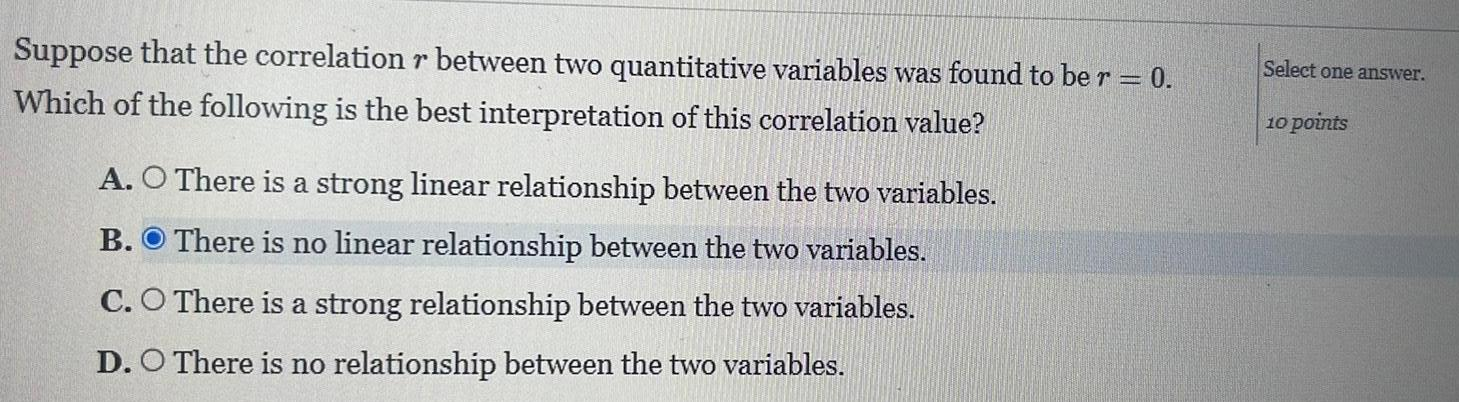Question:

# Suppose that the correlation r between two quantitative

Last updated: 9/13/2023Suppose that the correlation r between two quantitative variables was found to be r 0 Which of the following is the best interpretation of this correlation value A O There is a strong linear relationship between the two variables B There is no linear relationship between the two variables C O There is a strong relationship between the two variables D O There is no relationship between the two variables Select one answer 10 points